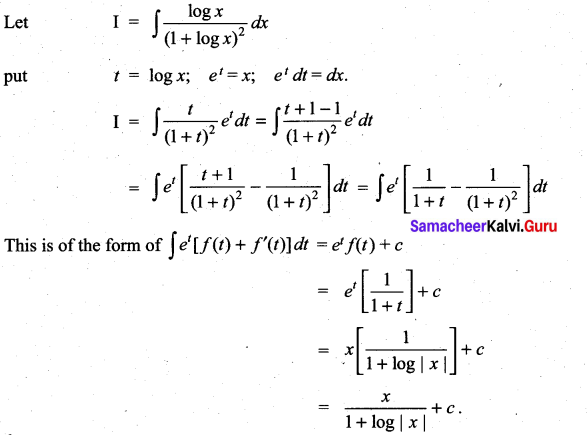## Tamilnadu Samacheer Kalvi 11th Maths Solutions Chapter 11 Integral Calculus Ex 11.9

Integrate the following with respect to x
Question 1.
ex (tan x + log sec x)
Solution: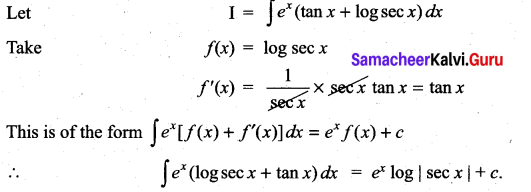Question 2.
ex$$\left(\frac{x-1}{2 x^{2}}\right)$$
Solution: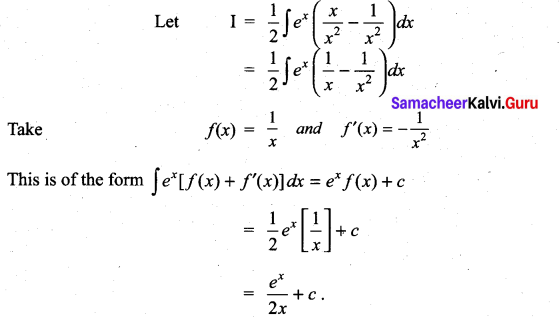Question 3.
ex sec x (1 + tan x)
Solution:
Let I = $$\mathrm{I}=\int e^{x}(\sec x+\sec x \tan x) d x$$
Take f(x) = sec x
f ‘ (x) = sec x tan x
This is of the form of $$\int e^{x}\left[f(x)+f^{\prime}(x)\right] d x$$ = ex f(x) + c
= ex sec x + cQuestion 4.
ex $$\left(\frac{2+\sin 2 x}{1+\cos 2 x}\right)$$
Solution: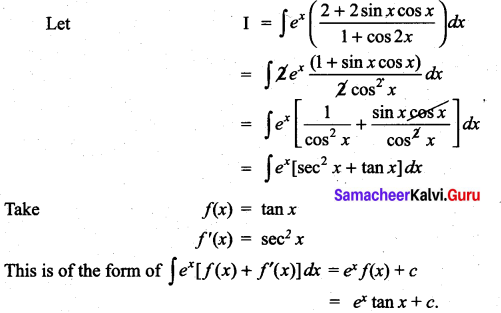Question 5.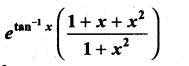Solution: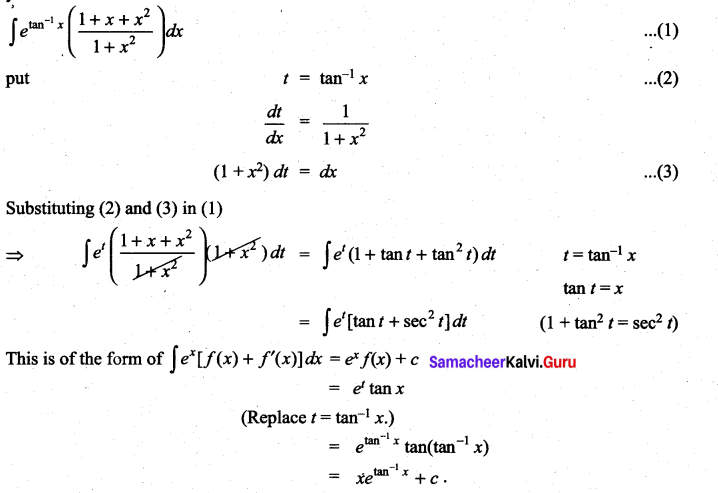Question 6.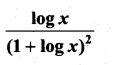Solution: